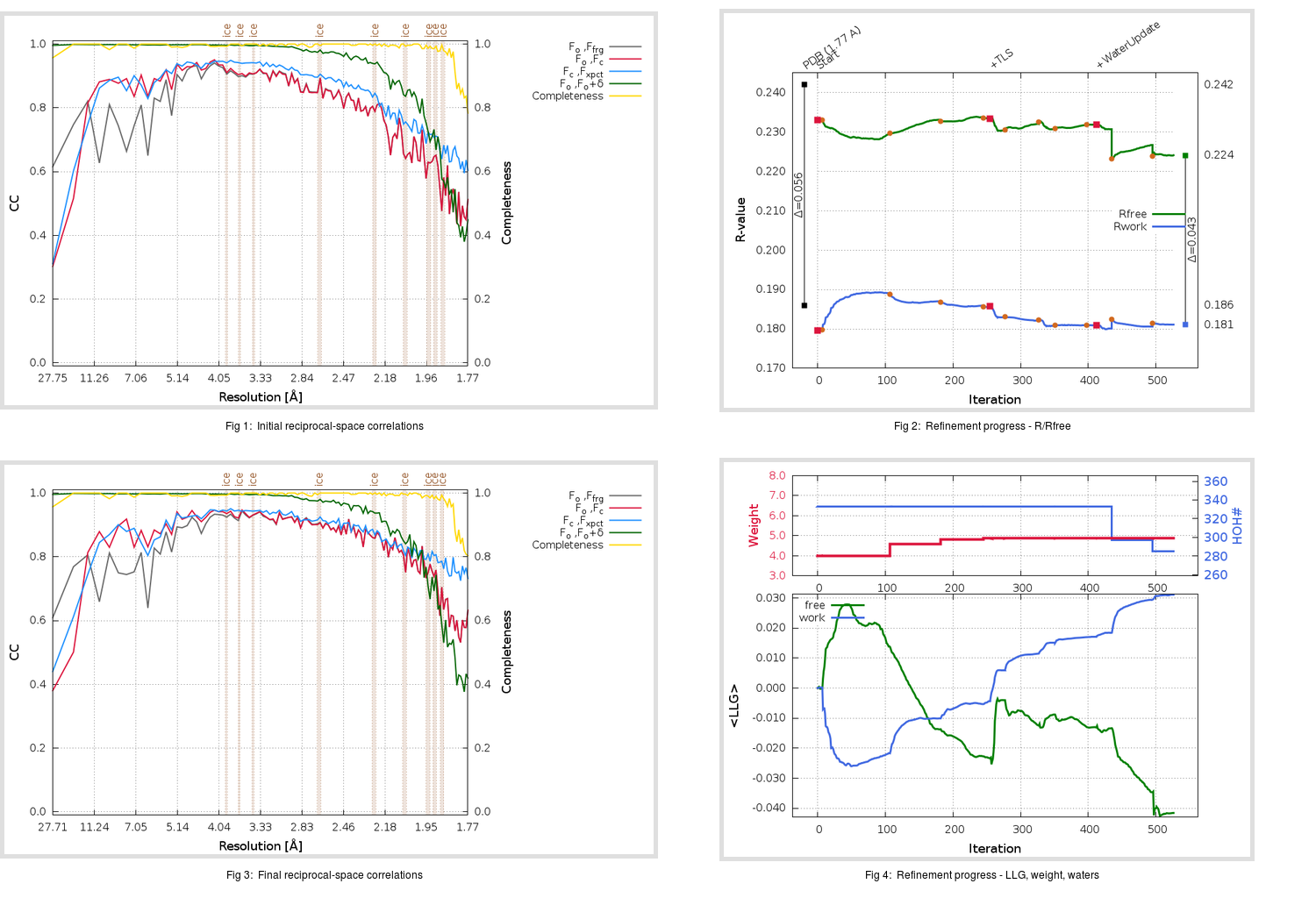Content:

```    Diffraction limits & principal axes of ellipsoid fitted to diffraction cut-off surface:
1.768         0.9570   0.0000   0.2902       a* + 0.030 c*
1.723         0.0000   1.0000   0.0000       b*
1.698        -0.2902   0.0000   0.9570      -0.596 a* + 0.803 c*
```

## Deposited

` `
 Date deposited Date data collection Resolution R, Rfree 20200315 20200227 1.77 0.1830 0.2420

Molprobity (CCP4 7.0 version) summary:

```Ramachandran outliers =   0.33 %
favored =  97.02 %
Rotamer outliers      =   0.76 %
C-beta deviations     =     1
Clashscore            =   6.34
RMS(bonds)            =   0.0131
RMS(angles)           =   1.83
MolProbity score      =   1.52
Resolution            =   1.77
R-work                =   0.1830
R-free                =   0.2420
```

```Number of waters      =   333

<B> (all atoms) =   29.94 ( sd =   11.15 ) for       2730 non-hydrogen atoms
<B>   (protein) =   28.35 ( sd =   10.28 ) for       2370 non-hydrogen atoms
<B>     (water) =   40.68 ( sd =   10.90 ) for        333 non-hydrogen atoms
<B>    (others) =   36.78 ( sd =   12.64 ) for         27 non-hydrogen atoms

B min/max       (all non-hydrogen atoms) =   15.24 /   88.64
B min/max   (protein non-hydrogen atoms) =   15.24 /   88.64
B min/max     (water non-hydrogen atoms) =   16.33 /   78.69
B min/max     (other non-hydrogen atoms) =   30.08 /   53.18
```

## BUSTER (re-)refinement

` `

Molprobity (CCP4 7.0 version) summary:

```Ramachandran outliers =   0.33 %
favored =  98.34 %
Rotamer outliers      =   1.52 %
C-beta deviations     =     0
Clashscore            =   2.96
RMS(bonds)            =   0.0114
RMS(angles)           =   1.57
MolProbity score      =   1.22
Resolution            =   1.77
R-work                =   0.1811
R-free                =   0.2241
```

```Number of waters      =   285

<B> (all atoms) =   30.95 ( sd =    9.35 ) for       2682 non-hydrogen atoms
<B>   (protein) =   29.65 ( sd =    8.46 ) for       2370 non-hydrogen atoms
<B>     (water) =   40.96 ( sd =    9.58 ) for        285 non-hydrogen atoms
<B>    (others) =   39.68 ( sd =   14.50 ) for         27 non-hydrogen atoms

B min/max       (all non-hydrogen atoms) =   16.25 /   70.15
B min/max   (protein non-hydrogen atoms) =   17.04 /   70.15
B min/max     (water non-hydrogen atoms) =   16.25 /   64.34
B min/max     (other non-hydrogen atoms) =   31.23 /   57.16
```

Refinement progression:Results:

` `
 File Remark 5REE_aB_refine.01_03_refine.pdb.gz exact refinement commands are in header 5REE_aB_refine.01_03_refine.mtz.gz including original deposited data and several re-refinement map coefficients 5REE_aB_refine.01_03_BUSTER_model.cif.gz including any non-standard compound restraints 5REE_aB_refine.01_03_BUSTER_refln.cif.gz